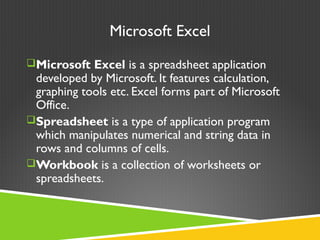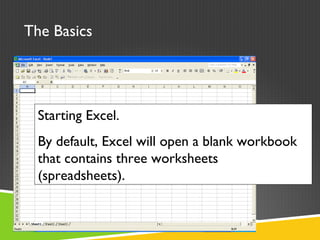Successfully reported this slideshow.

# Introduction to Microsoft Excel for beginners

26

Share×
1 of 36
1 of 36

# Introduction to Microsoft Excel for beginners

26

Share

Introduction to Microsoft Excel for beginners

Introduction to Microsoft Excel for beginners

## More Related Content

### Related Books

Free with a 14 day trial from Scribd

See all

### Related Audiobooks

Free with a 14 day trial from Scribd

See all

### Introduction to Microsoft Excel for beginners

1. 1. Introduction to Microsoft Excel
2. 2. Microsoft Excel Microsoft Excel is a spreadsheet application developed by Microsoft. It features calculation, graphing tools etc. Excel forms part of Microsoft Office. Spreadsheet is a type of application program which manipulates numerical and string data in rows and columns of cells. Workbook is a collection of worksheets or spreadsheets.
3. 3. The Basics Starting Excel. By default, Excel will open a blank workbook that contains three worksheets (spreadsheets).
5. 5. Toolbars
6. 6. Identify Excel components
7. 7. Descriptions of Excel components
8. 8. Microsoft Excel consists of worksheets. Each worksheet contains columns and rows. The columns are lettered A to IV; the rows are numbered 1 to 65536. The combination of column and row coordinates make up a cell address. For example, the cell located in the upper left corner of the worksheet is cell A1, meaning column A, row 1. Cell E10 is located under column E on row 10. You enter your data into the cells on the worksheet.
9. 9. A1 B3 E7
10. 10. Entering Data into a Worksheet  To enter data, first make the cell in which you want to enter the data active by clicking it.  Enter the data (text, formulas, dates, etc.) into the active cell.  Use the Alt+Enter key combination to enter text on multiple lines within the same cell.  UseTAB key, arrow keys, or ENTER key to navigate among the cells.
11. 11. Entering Data into a Worksheet
12. 12. Cells can be formatted to help handle various types of data. Right click on a single cell, or a group of cells, and select “Format Cells” from the drop down menu. Format CELLS
13. 13. Brief descriptions of format types can be seen at the bottom of the dialog box. Take a moment to look through the various formatting options.
14. 14. To change column width: Put cursor on line between two columns and drag to the right to the desired width
15. 15. Resize the columns so that you can see more of the data…
16. 16. Range selection techniques
17. 17. Sorting data is sometimes necessary. This data is currently in alphabetical order by last name.
18. 18. Filter In order to select a particular form of data from the numerous cells, we need to filter the data. Filtering helps us to separate the data we need from the data we do not need. To Filter the data, we have to click on the filter tab.
19. 19. To insert an additional worksheet inside this workbook, click on Insert/Worksheet.
20. 20. You can rename the new sheet by right clicking on its tab at the bottom, and selecting “Rename” from the menu.
21. 21. Copy/Cut & Paste: To copy/cut the data, highlight from cell through cell and copy the data. Paste it on the new sheet.
22. 22. Copy the Fall worksheet to the worksheets,Winter, Spring, and Summary 1. Click top of worksheet to select the entire worksheet 2. Click Copy icon
23. 23. 2. Click on Cell A1 3. Click Paste 4. Change the title to Winter Budget 1. Click Winter Tab 5. Follow the instructions to paste the Fall worksheet into the Spring and Summary Worksheets
24. 24. To draw charts and graphs, highlight the data you want to graphically represent. Then click the chart wizard icon on your toolbar. Charts and Graphs
25. 25. You can also launch the Chart Wizard by selecting Insert/Chart.
26. 26. Notice that the Chart toolbar appears when you insert a chart. You can edit the charts properties at any time.
27. 27. Formulas and Functions  Formulas are equations that perform calculations on values in your worksheet.A formula starts with an equal sign (=). For example, the following formula multiplies 2 by 3 and then adds 5 to the result. =5+2*3  Functions are predefined formulas that perform calculations by using specific values, called arguments, in a particular order, or structure. Functions can be used to perform simple or complex calculations.
28. 28. Looking at cell I19, we can see that there are no special functions associated with the data. Using a simple function can help make regular updates to the data easier.
29. 29. For example, if you click on the Autosum button while cell I19 is selected, Excel will identify the cells to include when calculating a total amount. Hit enter to lock the sum in. Notice that the function reads: =SUM(I12:I18)
30. 30. The same can be done using a formula, instead of a function. =I12+I13+I14+I15+I16+I17+I18 This long expression helps to show why functions are handy tools.
31. 31. Click on the small down arrow next to the function symbol (the epsilon). Select “More Functions.”
32. 32. Arithmetic Operators
33. 33. Excel Help
34. 34. Thank you